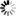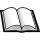Normal view

# Calculus : one-variable calculus, with an introduction to linear algebra, vol. 1 / Tom M. Apostol.

Material type:TextLanguage: English Publication details: New York : John Willey & Sons, c1991.Edition: 2nd edDescription: xx, 665 p. ; ill. : 27 cmISBN:
• 9780471000051
Subject(s): LOC classification:
• QA300 .A66 1991
Contents:
v. 1. One-variable calculus, with an introduction to linear algebra.--v. 2. Multi-variable calculus and linear algebra, with applications to differential equations and probability.
Tags from this library: No tags from this library for this title.
Star ratingsAverage rating: 0.0 (0 votes)
Holdings
Item type Current library Collection Shelving location Call number Vol info Copy number Status Date due BarcodeBooks North South University Library Non-fiction General Stacks QA300.A66 1991 (Browse shelf(Opens below)) vol- 1 1 Available 48630Books North South University Library Non-fiction General Stacks QA300.A66 1991 (Browse shelf(Opens below)) vol- 1 2 Available 48631
##### Browsing North South University Library shelves, Shelving location: General Stacks, Collection: Non-fictionClose shelf browser (Hides shelf browser)
 QA297.S33 1966 Numerical mathematical analysis / QA297.S33 1966 Numerical mathematical analysis / QA300.A66 1991 Calculus : one-variable calculus, with an introduction to linear algebra, vol. 1 / QA300.A66 1991 Calculus : one-variable calculus, with an introduction to linear algebra, vol. 1 / QA300.A66 1991 Calculus : Multi-variable calculus and linear algebra, with applications to differential equations and probability, vol. 2 / QA300.A66 1991 Calculus : Multi-variable calculus and linear algebra, with applications to differential equations and probability, vol. 2 / QA300.B37 2000 Introduction to real analysis /

Includes bibliographies & index.

v. 1. One-variable calculus, with an introduction to linear algebra.--v. 2. Multi-variable calculus and linear algebra, with applications to differential equations and probability.

Mathematics, Physics and Statistics

Sumaiya Kainat Bintey Kohinoor

There are no comments on this title.